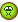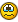## Recommended Posts

I have written this script to search for solutions to the equasion A^3 + B^3 = C^3; Where A, B and C are positive, whole integers. However, I am not very experianced at using arrays, and i am having some problems:

```:nuke:  :nuke:  :nuke:
Func calculate()
\$SUCCESS_RATE = 0
\$A = 0
\$B = 0
\$C = 0
\$QUITA = 0
\$QUITB = 0
\$AVALUES = 0
\$BVALUES = 0
While \$SUCCESS_RATE = 0
\$C = \$C + 1
\$CCUBED = \$C ^ 3
While \$QUITA = 0
Dim \$ACUBED[\$CCUBED - 1]
While \$AVALUES < \$CCUBED
\$AVALUES = \$AVALUES + 1
\$ACUBED[\$AVALUES] = \$AVALUES ^ 3
WEnd
WEnd
While \$QUITB = 0
Dim \$BCUBED[\$CCUBED - 1]
While \$BVALUES < \$CCUBED
\$BVALUES = \$BVALUES + 1
\$BCUBED[\$BVALUES] = \$BVALUES ^ 3
WEnd
WEnd
If \$ACUBED + \$BCUBED = \$CCUBED Then
\$SUCCESS_RATE = 1
EndIf
WEnd
GUICtrlSetData(\$EDIT_1, "- Success!" + @CRLF + "A=" + \$ACUBED^ (1 / 3) + @CRLF + "B=" + \$BCUBED^ (1 / 3) + @CRLF + "C=" + \$CCUBED^ (1 / 3))
EndFunc  ;==>calculate```Can anybody help?##### Share on other sites

Hello,

1) for the error, \$CCUBED-1=0 for the 1st time, so you are declaring an array with 0 as dimension and autoit doesn't like ...

so you can fixe it with this :

```While \$QUITA = 0
If \$C<>1 Then
Dim \$ACUBED[\$CCUBED-1]
While \$AVALUES < \$CCUBED
\$AVALUES = \$AVALUES + 1
\$ACUBED[\$AVALUES] = \$AVALUES ^ 3
WEnd
EndIf
WEnd```

2) You'll never exit from this loop because \$QUITA will never change in this loop

3) x^n+y^n=z^n has no positive integer solution (x,y,z) with n>2 (Ferma's theorem)

##### Share on other sites

Thanks, the "while \$QUITA = 0 ; Wend" was a mistake, and the script is only intended for demonstration purposes, but thanks for the help with the arrays

## Create an account

Register a new account

• ### Recently Browsing   0 members

×

• Wiki

• Back

• #### Beta

• Git
• FAQ
• Our Picks
×
• Create New...